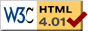### Journée-séminaire de combinatoire

#### (équipe CALIN du LIPN, université Paris-Nord, Villetaneuse)

Le 05 avril 2011 à 10h30 en B311, Aristide Baratin nous parlera de : Combinatorial state sum invariants from categories

Résumé : "Combinatorial state sum invariants from categories"
'State sum models' are discrete functional path integrals. Using the combinatorics of the Pachner moves of the triangulation to convert a topological problem into an algebraic one, these models can be used to define manifold invariants and topological quantum field theories. Just as 3d state sum models, including quantum gravity, can be built using categories of group representations, 2-categories of 2-group representations may provide interesting state sum models for 4d quantum topology, if not quantum gravity. I will describe the construction of the first non-trivial example of a such models, based on the representations of a categorical version of the Euclidean group. I will show that this model shows up naturally in a combinatorial (state sum) reformulation of Feynman integrals for ordinary quantum field theories on 4d Euclidean spacetime.

 Dernière modification : Monday 24 January 2022Contact pour cette page : Cyril.Banderier at lipn.univ-paris13.fr